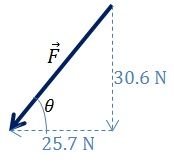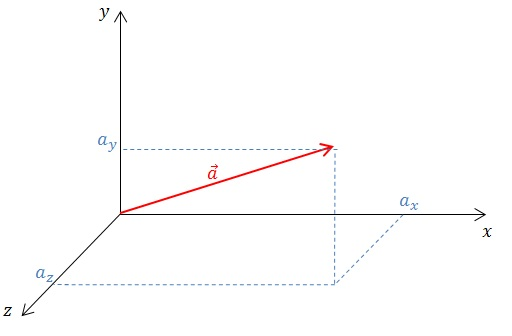# What is a Vector

## Definition of a Vector

A vector is a quantity with both a magnitude (size) and direction. Geometrically, a vector can be represented by a directed line segment, whose direction points in the direction of the vector and whose length is proportional to the magnitude of the vector.

## How to Write a Vector

A vector can be written down in several ways. One method is to use bold characters e.g.$\textbf{a}$. You can also use an underline ($\underline{a}$) or an arrow drawn on top of a letter ($\vec{a}$). If the symbol for a vector is written without these, it is taken to be the magnitude of the vector.

Two vectors that have the same length and direction are equal. In the diagram below,$\vec{p} = \vec{q}$.## How to Find Components of a Vector

To find the component of a vector in a given direction, draw a line parallel to the needed direction, passing through the “tail” end of the vector. Then, drop a perpendicular line from the “nose” of the vector onto this line. The component of the vector in the given direction is, then, the length of the line from the “tail” of the vector to the dropped perpendicular line.

For example, on the diagram below, the component of the vector$\vec{a}$ along the$x$-axis is$a_x$ and the component along the$y$-axis is$a_y$From trigonometry, we have:$a_x = a\textup{cos} \theta$ and,$a_y = a\textup{cos} (90^o-\theta) = a\textup{sin} \theta$

Generally, if a vector with magnitude$a$ makes an angle$\theta$ to a given direction, then the component of the vector along that direction is$a\textup{cos}\theta$, and the component of the vector in the direction perpendicular to that direction is$a\textup{sin}\theta$.

Example

An aeroplane takes off at a speed of 253 km h-1, making an angle of 15o to the runway. Assuming the Sun is shining directly overhead, find the speed of the aeroplane’s shadow along the runway.

The speed of the shadow is the component of the plane’s velocity along the runway. Since the plane is travelling at an angle of 15o to the runway, the speed of the shadow is then$253\times \textup{cos}15^o=244$ km h-1.

Conversely, if components of a vector along two perpendicular directions are known, we can use simple trigonometry to find the angle that the vector makes along one of the directions, and we can also calculate the size of the original vector.

Example

A lawnmower is pushed along the ground, with a force$\vec{F}$ exerted along the handle. The vertical and horizontal components of the force are 30.6 N and 25.7 N respectively. Find a) size of the force$\vec{F}$ and b) the angle$\theta$ that the lawnmower makes with the ground.Firstly, to find the size of the force, we use the Pythagoras’ theorem:$F=\sqrt{30.6^2+25.7^2}\approx \sqrt{1600}\approx 40$ N.

The angle$\theta$ is given by$\theta =\textup{tan}^{-1}\left (\frac{30.6}{25.7}\right )\approx 50^o$

## How to Represent Vectors in the Cartesian Coordinate System

If the components of a vector$\vec{a}$ along the$x$$y$ and$z$ axes are$a_x$$a_y$ and$a_z$ respectively, the vector can be written as$\vec{a}=(a_x,a_y,a_z)$.## How to find the Magnitude of a Vector

Magnitude refers to the size of the vector, without taking into consideration its direction. The magnitude of a vector$\vec{a}$ is written as$\left|\vec{a}\right|$. If the letter is simply written as$a$, this is also taken to indicate the magnitude of the vector.

If a vector$\vec{a}=(a_x,a_y,a_z)$, then its magnitude$\left|\vec{a}\right| = \sqrt{{a_x}^2+{a_y}^2+{a_z}^2}$.

Example

The electric field vector at a point is given by$\vec{E} = (-0.8, 2.3,1.5)$ N C-1. Find the magnitude of the electric field.$\left|\vec{E}\right| = \sqrt{{(-0.8)}^2+{2.3}^2+{1.5}^2} \approx 3$ N C-1.

## What are Unit Vectors

A unit vector is a vector with a magnitude of 1 unit. Unit vectors are often written with a ‘hat’ above the letter. e.g.$\hat{a}$. The unit vector along the direction of a vector$\vec{a}$, is defined as:$\hat{a} =\frac{\vec{a}}{\left|\vec{a}\right|}$

In particular, on the Cartesian coordinate system, the unit vectors along the$x$$y$ and$z$ axes are written as$\hat{i}$,$\hat{j}$ and$\hat{k}$ respectively.

Using these unit vectors, a vector in the 3-dimensional Cartesian coordinate system can be written as a sum of 3 vectors along the$x$$y$ and$z$ directions. This is done by taking components of the vector along$x$$y$ and$z$ axes, and multiplying each component by the unit vector of the corresponding axis.

For example, the vector$\vec{a}=(a_x,a_y,a_z)$ can be written as$\vec{a}=a_x\hat{i}+a_y\hat{j}+a_z\hat{k}$.

## How to Add and Subtract Vectors